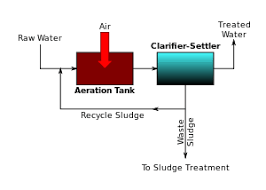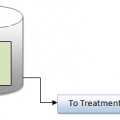## How to Calculate Aeration Tank Volume?Dear reader, due to some heavy work.we couldn’t touch with u last few months, OK.

Now we see as an with small example!

Aeration tank:

Influent BOD: 250mg/l(after 30% reduction in the Physio-chemical treatment followed by primary clarifier

Process selected: Activated sludge process

Design Condition:

F/M (Food/Microbes) =0.15

MLSS (Mixed Liquor Suspended Solids)=3000 mg/l

Calculation of Aeration Tank Volume:

F/M: So (?-MLVSS)

F/M: Food to Microbes ratio in d-1

So: influent substrate concentration in mg/l

MLVSS: Mixed Liquor Volatile Suspended solids in mg/l =0.8 x MLSS

? – Hydraulic retention time =V/Q

Where V=Aeration tank volume in cu.m

Q=Effluent flow in cu.m per day

Hence, substituting these values,

0.15=250/(v/1400 x 0.8 x 3000)

V=972 KL, selected volume =1400 KL## Author:T.P.Sivanandan

#### Why Fine Bubbles are Better than Coarse bubble in Aeration Tank#### How to Calculate Lime Dosage Requirement in Sedimentation Process## 18 thoughts on “How to Calculate Aeration Tank Volume?”

1.Jaime G. Nagac says:

I have just read your topic on aeration volume calculation today. It’s simple and very interesting to me but I would like to know where did you get the number 1400 in your formula or equation? 0.15= 250/(v/1400×0.8×3000). Thank you.

1.Vidit Arya says:

Exactly, The information is valuable, Until, eventually the number 1400 was found unknown.

Acc. to the formula I use to calc. the Volume is
V= Q.BOD / (F/M).MLSS

And my question is that how will be the volume of the MBBR based aeration can be calculated?

2.N.balamurugan says:

Dear Sir
Every think OK.but I don’t know about the value of 1400. For which using that one

1.T.P.Sivanandan says:

1400 is assumption fig of water effluent quantity in M3/day

3.r.vijaykumar says:

hai sir
i need differnt example on areartion tank design, can you give me .

4.S.sankar says:

Where did you get value 1400 in your formula and v express to what?

1.T.P.Sivanandan says:

resolved check the post

5.Pankaj D Thakkar says:

1400 is plant capacity I.e. You want to handle 1400 m3 per day. This can be as per your need.
e.g. I am going to establish Etp plant of 900 m3 per day then Q= 900 m3/ day Accordingly Vaerationn will be less.

6.SAQIB HAMID says:

Design an aeration tank for a flow of 790 m3/ hour with BOD of 200 mg/L an F/M ratio o f0.4 day-1 and SVI of 100 ml/gram. Air supply rate is 10 m3/ m2 of sewage /day. Assume W: L = 1:4.
PLEASE IF ANY ONE CAN SOLVE THIS

7.mathankumar says:

Explain the equation sir.

8.joseph Howeiss says:

i cant understand the KL the volume tank =972 KL ITS MEAN 972,000LITERS?

1.T.P.Sivanandan says:

KL mean Kilo Litre (1000 Litre)

9.joseph Howeiss says:

how we calculate the volume didnt arrive to find v

10.Akshay Dedge says:

Dear sir,
Thanks for the example..it was realy very helpful
But i Didnt understood the last equation..from where did u get v=972?
Pls someone explain..

1.T.P.Sivanandan says:

V mean Volume of Aeration Tank in m3 (in capacity of m3 holding) and 1400 mean treatment qty to be treated /day

11.Rup mishra says:

Sir, my capacity tank has a capacity of 480 kld/per day so how much capacity will be the air blower?The tank has to be lane in the air grid.

12.Vinod says:

What is the basics of considering F/M ratio – 0.15, MLSS – 3000 mg/lit? How it was link with COD And BOD? Or Its Just assumptions.

13.S.K.Deshpande says: